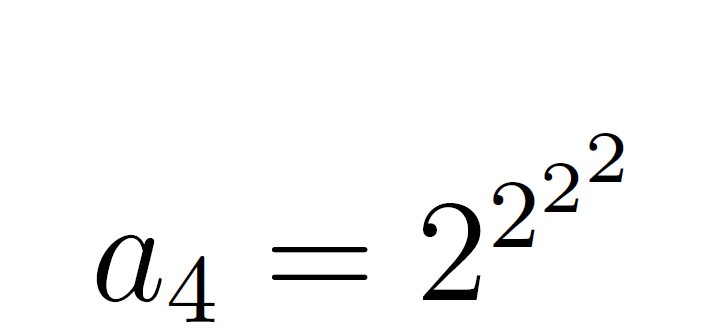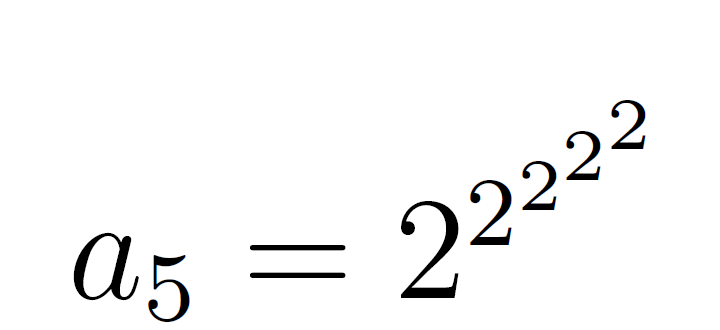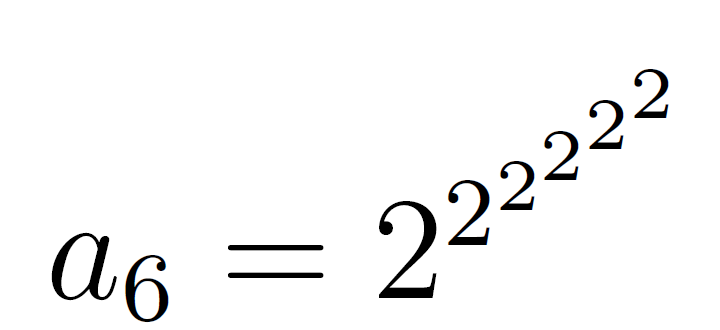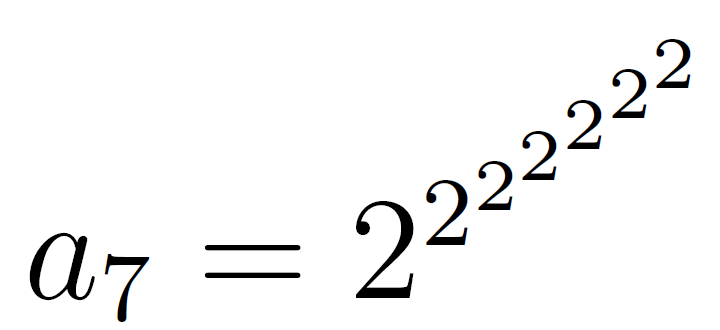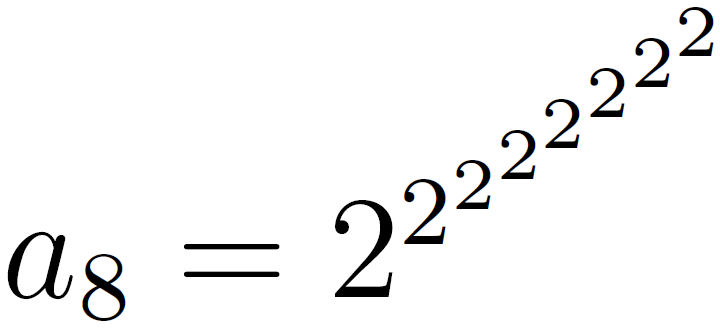## amc-2014-sr-25

The sequence

$2, 2^2, 2^{2^2}, 2^{2^{2^2}}, \ldots %speech% , 2. 2 to the power of 2. 2 to the power of 2 to the power of 2, and so on,$

is defined by $$a_1=2 %speech% ,aywon equals 2,$$ and $$a_{n+1}=2^{a_n} %speech% ,a n plus 1 equals 2 to the power of a n,$$ for all $$n\geq 1 %speech% ,n greater than or equal to 1,$$.

What is the first term in the sequence greater than $$1000^{1000} %speech% ,1000 to the power of 1000,$$?